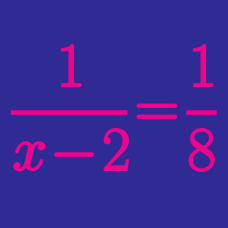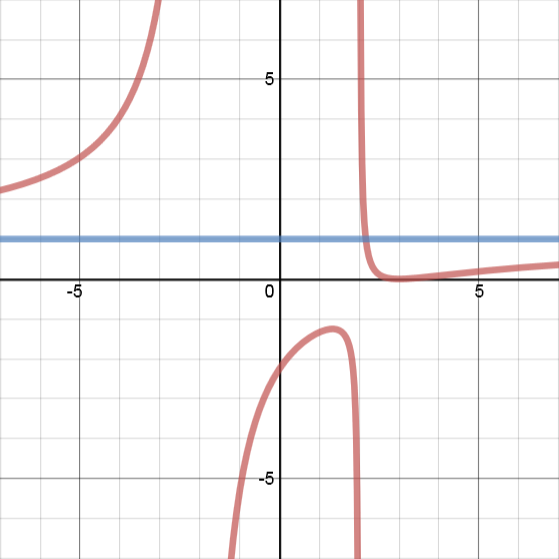Algebra

# Rational Functions Warmup

What is the y-intercept of the graph of $y=\dfrac{(x+3)(x-8)}{(x-6)(x+2)}$

$\dfrac{(2x+2)}{(x+2)(x+7)} = 1$ What are the possible values of $x$ ?

What is the range of the function defined by $f(x) = \dfrac{(x+2)(x+2)(x+2)}{(x+2)} ?$The red line above is a graph of $y=f(x).$ The graph travels through the points $(3,0)$ and $(0, -2.25).$

The blue line shows the horizontal asymptote of $f(x)$.

What is a possible definition for $f(x)$?

Where are the vertical asymptotes of the function $f(x) = \dfrac{(x-6)(x-4)}{2(x-4)(x+3)}$ ?

×